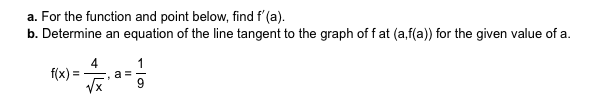# a. For the function and point below, find f'(a) b. Determine an equation of the line tangent to the graph of f at (a,f(a)) for the given value of a f(x)

Questionhelp_outlineImage Transcriptionclosea. For the function and point below, find f'(a) b. Determine an equation of the line tangent to the graph of f at (a,f(a)) for the given value of a f(x) fullscreen

### Want to see the step-by-step answer?